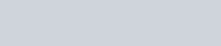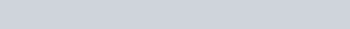# Water horse power (whp.)

WATER HORSE POWER (WHP.) W003

The calculated water power or work done by the pump.

Here is the formula for the horsepower:In SI units (metric) we make the calculations in kilowatts:If you divide the water horsepower by the pump’s brake horse power you get the pump’s efficiency.

### Posted

• On February 17, 2018